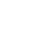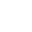> > Equation of Hyperbola

# Equation of Hyperbola

A hyperbola is the set of all points in a plane, the difference of whose distances from two fixed points in the plane is constant. ‘Difference’ means the distance to the ‘farther’ point minus the distance to the ‘closer’ point. The two fixed points are the foci and the mid-point of the line segment joining the foci is the center of the hyperbola.

The line through the foci is called the transverse axis. Also, the line through the center and perpendicular to the transverse axis is called the conjugate axis. The points at which the hyperbola intersects the transverse axis are called the vertices of the hyperbola.• The distance between the two foci is: 2c
• The distance between two vertices is: 2a (this is also the length of the transverse axis)
• The length of the conjugate axis is 2b … where b = √ (c2 – a2)

### Suggested VideosIntroduction to Conic SectionsTerms Associated to ConicsDiameter of a Parabola## Finding the Constant P1F2 – P1F1

Refer the diagram below:

##We take a point P at A and B as shown above. Therefore, by the definition of a hyperbola, we have

BF1 – BF2 = AF2 – AF1
∴ BA + AF1 – BF2 = AB + BF2 – AF1

Solving the equation, we get, AF1 = BF2
Hence, BF1 – BF2 = BA + AF1 – BF2 = BA = 2a.### Eccentricity

Like in the ellipse, e = c/a is the eccentricity in a hyperbola. Also, ‘c’ is always greater than or equal to ‘a’. Hence, the eccentricity is never less than one.

## Standard Equation of Hyperbola

When the center of the hyperbola is at the origin and the foci are on the x-axis or y-axis, then the equation of the hyperbola is the simplest. Here are two such possible orientations:Of these, let’s derive the equation for the hyperbola shown in Fig.3 (a) with the foci on the x-axis. Let F1 and F2 be the foci and O be the mid-point of the line segment F1F2.

Also, let O be the origin and the line through O through F2 be the positive x-axis and that through F1 as the negative x-axis. The line through O perpendicular to the x-axis be the y-axis. Let the coordinates of F1 be (– c,0) and F2 be (c,0) shown in Fig. 3 (a).

## Derivation of the EquationNow, we take a point P(x, y) on the hyperbola such that, PF1 – PF2 = 2a

By the distance formula, we have,
√ {(x + c)2 + y2} – √ {(x – c)2 + y2} = 2a
Or, √ {(x + c)2 + y2} = 2a + √ {(x – c)2 + y2}

Further, let’s square both the sides. Hence, we have
(x + c)2 + y2 = 4a2 + 4a√ {(x – c)2 + y2} + (x – c)2 + y2

On simplifying the equation, we get
√ {(x – c)2 + y2} = x(c/a) – a

We square both sides again and simplify it further to get,
x2/a2 – y2/(c2 – a2) = 1

We know that c2 – a2 = b2. Therefore, we have
x2/a2 – y2/b2 = 1

Therefore, we can say that any point on the hyperbola satisfies the equation:

x2/a2 – y2/b2 = 1 … (1)

### Converse situation

Let’s look at the converse situation now. If P(x, y) satisfies equation (1) with 0 < a < c, then
y2 = b2{(x2 – a2)/a2}

Therefore, PF1 = √ {(x + c)2 + y2}
= √ {(x + c)2 + b2[(x2 – a2)/a2])}

On simplifying the equation, we get PF1 = a + x(c/a)
Using similar calculations for PF2, we get PF2 = a – x(c/a)

In hyperbola c > a; and since P is to the right of the line x = a, x > a, and (c/a)x > a. Therefore, a – (c/a)x becomes negative.
Thus, PF2 = (c/a)x – a
Therefore, PF1 – PF2 = {a + x(c/a)} – {x(c/a) – a} = a + x(c/a) – x(c/a) + a = 2a.

Also, note that if P is to the left of the line x = – a, then,
PF1 = – {a + (c/a)x} and PF2 = a – (c/a)x
In this case, PF1 – PF2 = 2a.
Hence, it is evident that any point that satisfies the equation x2/a2 – y2/b2 = 1, lies on the hyperbola.

#### Note

Solving the equation, we get

x2/a2 = 1 + y2/b2 ≥ 1

Therefore, no portion of the curve lies between the lines x = + a and x = – a. Similarly, we can derive the equation of the hyperbola in Fig. 3 (b) as

y2/a2 – x2/b2 = 1

These two equations are known as the Standard Equations of Hyperbolas.

### Observations

• A hyperbola is symmetric with respect to both the coordinate axes. In simple words, if (m, n) is a point on the hyperbola, then (- m, n), (m, – n) and (- m, – n) also fall on it.
• The foci always lie on the transverse axis. The denominator of the positive term gives the transverse axis. For e.g. the transverse axis of x2/9 – y2/16 = 1 is along the x-axis and has length = 2a = 2 x √9 = 2 x 3 = 6.

## Latus rectum of Hyperbola

Latus rectum of a hyperbola is a line segment perpendicular to the transverse axis through any of the foci and whose endpoints lie on the hyperbola. The length of the latus rectum in hyperbola is 2b2/a.

## Solved Problems for You

Question 1: Find the equation of the hyperbola where foci are (0, ±12) and the length of the latus rectum is 36.

Answer: The foci are (0, ±12). Hence, c = 12. Length of the latus rectum = 36 = 2b2/a

∴ b2 = 18a

Hence, from c2 = a2 + b2, we have 122 = a2 + 18a
Or, 144 = a2 + 18a
i.e. a2 + 18a – 144 = 0
Solving it, we get a = – 24, 6

Since ‘a’ cannot be negative, we take a = 6 and so b2 = 36a/2 = (36 x 6)/2 = 108. Therefore, the equation of the required hyperbola is y2/36 – x2/108 = 1.

Question 2: What is the focus of hyperbola?

Answer: The foci of a hyperbola are the two fixed points which are situated inside each curve of a hyperbola which is useful in the curve’s formal definition.

Question 3: What is the difference between a parabola and a hyperbola?

Answer: A parabola comprises of two arms of the curve which we also refer to as branches that become parallel to each other. However, in a hyperbola, the two arms or curves do not become parallel.

Question 4: What makes a hyperbola?

Answer: An intersection of a plane perpendicular to the bases of a double cone forms a hyperbola. All the hyperbolas have two branches having a vertex and focal point. Moreover, all hyperbolas have an eccentricity value which is greater than 1.

Question 5: What is the use of hyperbola?

Answer: We see that the hyperbolic paraboloid is a 3D surface that is a hyperbola in one cross-section and a parabola in the other. Thus, we make use of hyperbolic structures in Cooling Towers of Nuclear Reactors.

Share with friends

## Customize your course in 30 seconds

##### Which class are you in?
5th
6th
7th
8th
9th
10th
11th
12th
Get ready for all-new Live Classes!
Now learn Live with India's best teachers. Join courses with the best schedule and enjoy fun and interactive classes.Ashhar Firdausi
IIT Roorkee
BiologyDr. Nazma Shaik
VTU
ChemistryGaurav Tiwari
APJAKTU
Physics
Get Started

## Browse

##### Conic Sections2 Followers

Most reacted comment
1 Comment authorsRecent comment authors
Subscribe
Notify ofGuest
Dami

Nigeria not among

## Question Mark?

Have a doubt at 3 am? Our experts are available 24x7. Connect with a tutor instantly and get your concepts cleared in less than 3 steps.